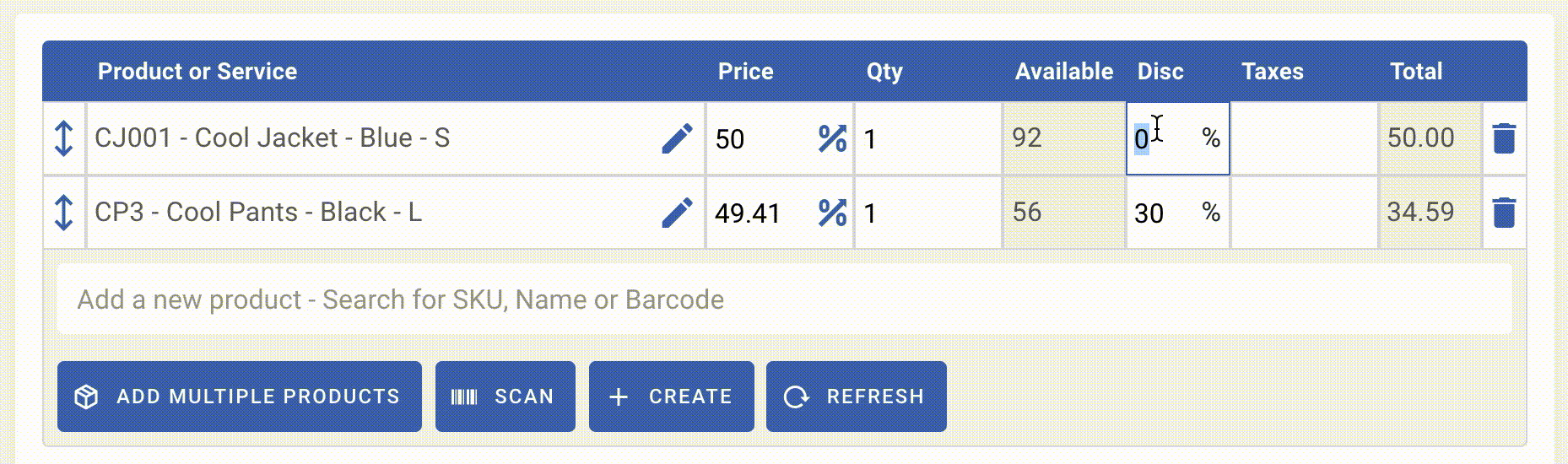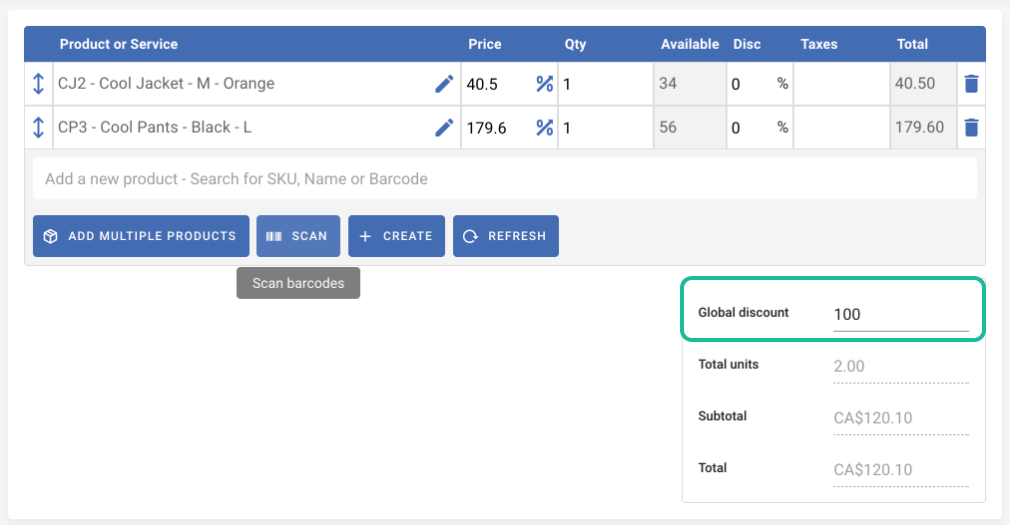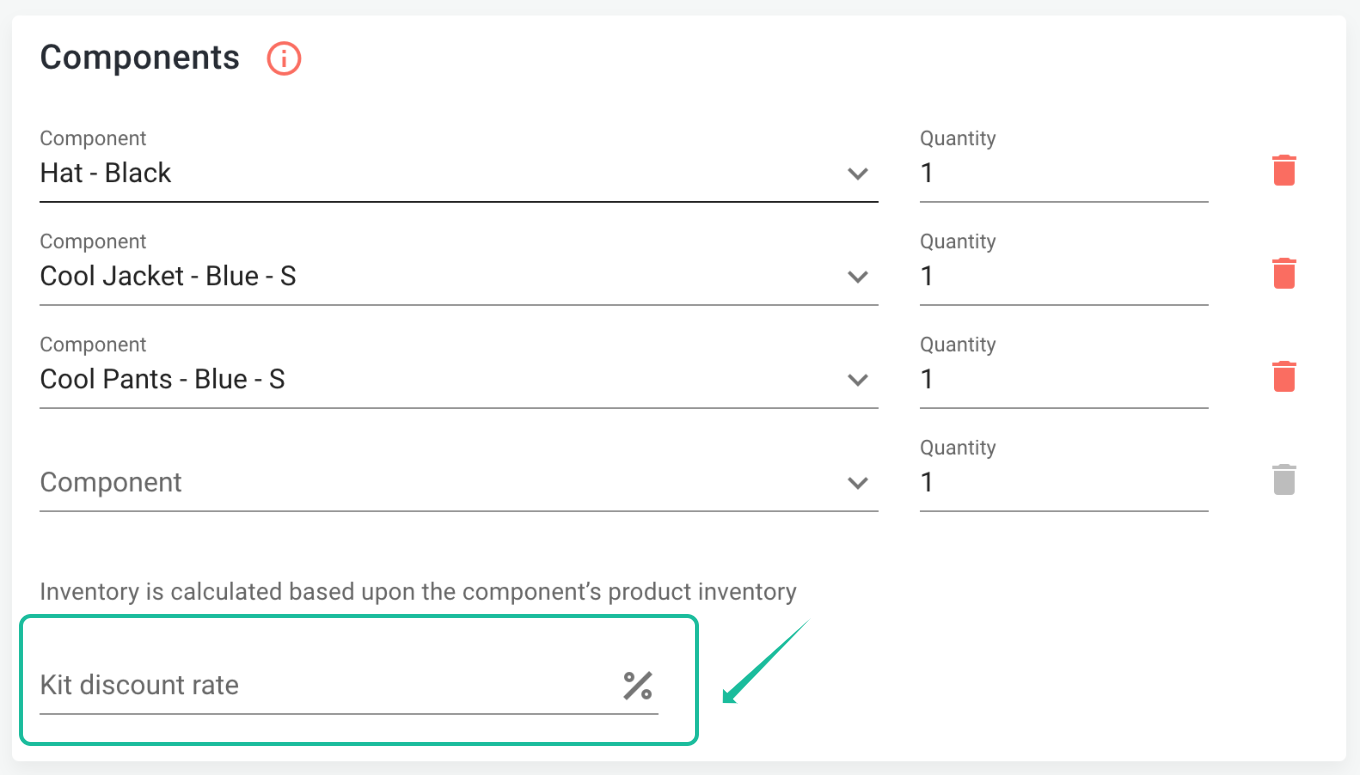# Discounts

## Applying discounts

There are two methods to apply discounts in your sales.

1. Discounted items

In each line item, use the column "Disc" to apply a percentage "%" or cash "\$" discount on your products.2. Global discount

At the bottom of the page, use the field "global discount" to apply a discount over the sale. (cash discount only).The global discount is distributed across each line item, proportionally, as follows:

The first line item represents 18,4% of the sale's total amount.
This line is discounted by an amount equal to 18,4% of the global discount. (100 x 0,184 = 18,4)
Product price after discount: (40,50 - 18,40 = \$22,10)

The second line item represents 81,6% of the sale's total amount
This line is discounted by an amount equal to 81,6% of the global discount.  (100 x 0,816 = 81,6)
Product price after discount: (179,6 - 81,6 = \$98)

TOTAL INCLUDING DISCOUNT: 98 + 22,10 = \$120.10

## Discounts and kits

When creating or editing kits, discounts can be applied on kits. In this case, the discount will apply on all components, individually.Example:

If a kit, discounted by 10% is made of one product sold at \$25 and a second product sold at \$40, here is how the discounts would calculate:

- Discount on first component: 25 x 10 / 100 = \$2,5
- Discount on second component: 40 x 10 / 100 = \$4

To learn more about kits, we recommend you to check the article Assemblies and Kits (New version).

Did you find it helpful? Yes No

Send feedback